##### Managerial Economics For Dummies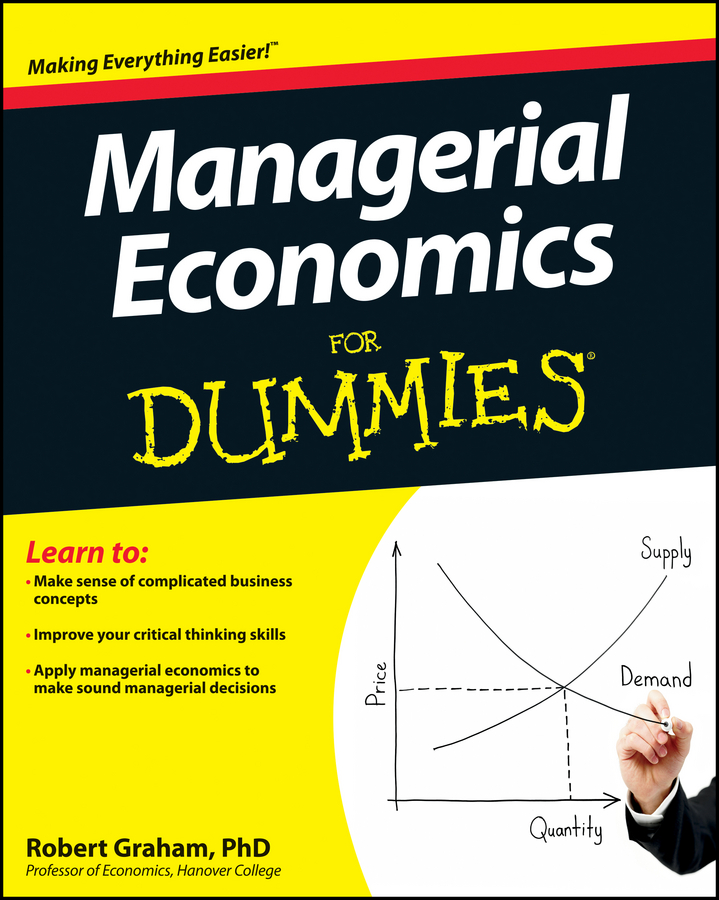Business situations are further complicated by constraints, which can be accounted for in managerial economics using the Lagrangian function. Perhaps the business has signed a contract to produce 1,000 units of the good daily, or the business has certain inputs, such as the factory size, that can’t be changed. Constraints limit the firm’s options. Your goal is to optimize a function subject to the limitations or constraints.

The Lagrangian function is a technique that combines the function being optimized with functions describing the constraint or constraints into a single equation. Solving the Lagrangian function allows you to optimize the variable you choose, subject to the constraints you can’t change.

## How to identify your objective (function)

The objective function is the function that you’re optimizing. The dependent variable in the objective function represents your goal — the variable you want to optimize. Examples of objective functions include the profit function to maximize profit and the utility function for consumers to maximize satisfaction (utility).

## Constraint functions

A constraint function represents a limitation on your behavior. The dependent variable in the constraint represents the limitation. Examples of constraint functions include the number of units you must produce in order to satisfy a contract and the budget available to a consumer.

## How to construct the Lagrangian function

The technique for constructing a Lagrangian function is to combine the objective function and all constraints in a manner that satisfies two conditions. First, optimizing the Lagrangian function must result in the objective function’s optimization. Second, all constraints must be satisfied. In order to satisfy these conditions, use the following steps to specify the Lagrangian function.

Assume u is the variable being optimized and that it’s a function of the variables x and z. Therefore,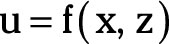In addition, there are two constraints, c1 and c2, that are also functions of x and z;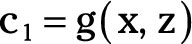The following steps establish the Lagrangian function:

1. Respecify the constraints so that they equal zero.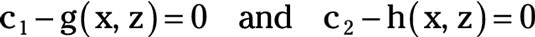2. Multiply the constraints by the factors lambda one and lambda two, ë1 and ë2, respectively (more on these in a moment).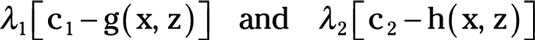3. Add the constraints with the lambda term to the objective function in order to form the Lagrangian function Â’.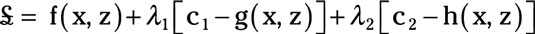In this specification of the Lagrangian function, the variables are represented by x, z, λ 1, and λ 2. Taking the partial derivatives of the Lagrangian with respect to λ 1 and λ 2 and setting them equal to zero ensure that your constraints are satisfied, while taking the partial derivatives of the Lagrangian with respect to x and z and setting them equal to zero optimize your objective function.

## The Lagrangian Multiplier

Managerial economics has a lot of useful shortcuts. One of those shortcuts is the λ used in the Lagrangian function. In the Lagrangian function, the constraints are multiplied by the variable λ, which is called the Lagrangian multiplier.

This variable is important because λ measures the change that occurs in the variable being optimized given a one-unit change in the constraint. If you’re trying to minimize the cost of producing a given quantity of output, λ tells you how much total cost changes if you produce one more unit of output. This enables you to quickly assess the relationships between constraints and the variable being optimized.

Suppose that your firm has a contract that requires it to produce 1,000 units of a good daily. The firm uses labor and capital to produce the good. The quantity of labor employed, L, is measured in hours, and the wage is \$10 per hour. The quantity of capital employed, K, is measured in machine-hours, and the price per machine hour is \$40. Thus, your firm’s total cost, TC, equals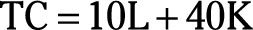The production function describes the relationship between the amounts of labor and capital used and the quantity of the good produced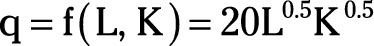By contract, q must equal 1,000. You must determine the amount of labor and capital to use in order to minimize the cost of producing the 1,000 units of the good.

1. Create a Lagrangian function. Recognize that the variable you’re trying to optimize is total cost — specifically, you’re trying to minimize total cost. So, your objective function is 10L + 40K. Second, your constraint is that 1,000 units of the good have to be produced from the production function. So your constraint is

1,000 – 20L0.5K0.5 = 0.

Your Lagrangian function is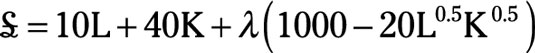2. Take the partial derivative of the Lagrangian with respect to labor and capital — L and K — and set them equal to zero. These equations ensure that the objective function is being optimized — in this case, total cost is minimized.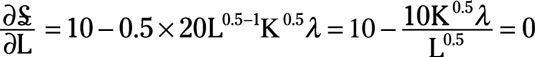3. Take the partial derivative of the Lagrangian function with respect to ë and set it equal to zero. This partial derivative ensures that the constraint — producing 1,000 units of the good daily — is satisfied.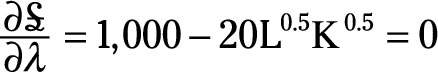4. Solve the three partial derivatives simultaneously for the variables L, K, and ë to minimize the total cost of producing 1,000 units of the good.

Rewriting the partial derivative of Β’ with respect to L enables you to solve for λ.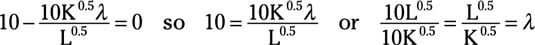Substituting the previous equation for λ in the partial derivative of Β’ with respect to K yields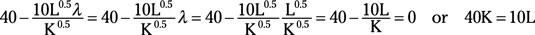5. Substitute 4K for L in the constraint (the partial derivative of L with respect to ë) to yield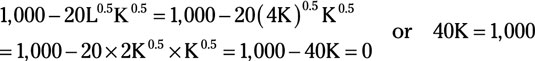Thus, your firm should use 25 machine hours of capital daily.

Because you earlier determined L = 4K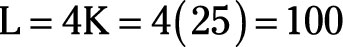Finally, you can solve for λ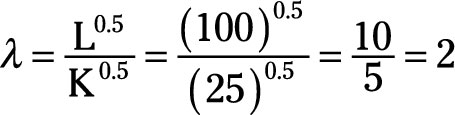Therefore, the combination 100 hours of labor and 25 machine-hours of capital minimize the total cost of producing 1,000 units of the good daily. In addition, λ equals 2. Remember that lambda indicates the change that occurs in the objective function given a one unit change in the constraint. Thus, if your firm wants to produce one more unit of the good, your total cost increases by \$2.